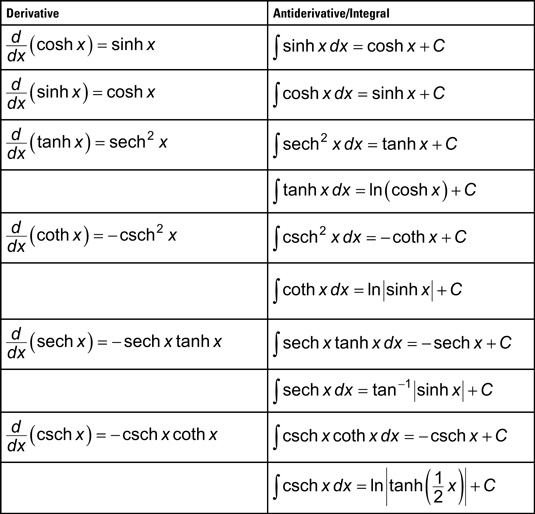##### Calculus: 1001 Practice Problems For Dummies (+ Free Online Practice)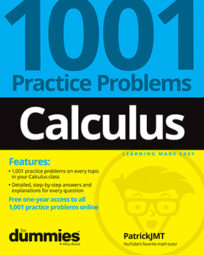Solving calculus problems is a great way to master the various rules, theorems, and calculations you encounter in a typical Calculus class. This Cheat Sheet provides some basic formulas you can refer to regularly to make solving calculus problems a breeze (well, maybe not a breeze, but definitely easier).

## Useful calculus theorems, formulas, and definitions

Following are some of the most frequently used theorems, formulas, and definitions that you encounter in a calculus class for a single variable. The list isn’t comprehensive, but it should cover the items you’ll use most often.

## Limit Definition of a Derivative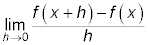## Definition: Continuous at a number a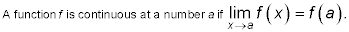## The Intermediate Value Theorem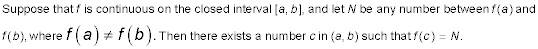## Definition of a Critical Number

A critical number of a function f is a number c in the domain of f such that either f (c) = 0 or f (c) does not exist.

## Rolle’s Theorem

Let f be a function that satisfies the following three hypotheses:

• f is continuous on the closed interval [a, b].

• f is differentiable on the open interval (a, b).

• f(a) = f(b).

Then there is a number c in (a, b) such that f (c) = 0.

## The Mean Value Theorem

Let f be a function that satisfies the following hypotheses:

• f is continuous on the closed interval [a, b].

• f is differentiable on the open interval (a, b).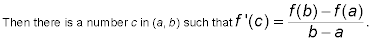## Newton’s Method Approximation Formula

Newton’s method is a technique that tries to find a root of an equation. To begin, you try to pick a number that’s “close” to the value of a root and call this value x1. Picking x1 may involve some trial and error; if you’re dealing with a continuous function on some interval (or possibly the entire real line), the intermediate value theorem may narrow down the interval under consideration. After picking x1, you use the recursive formula given here to find successive approximations: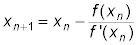A word of caution: Always verify that your final approximation is correct (or close to the value of the root). Newton’s method can fail in some instances, based on the value picked for x1. Any calculus text that covers Newton’s method should point out these shortcomings.

## The Fundamental Theorem of Calculus

Suppose f is continuous on [a, b]. Then the following statements are true: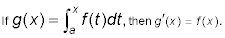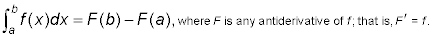## The Trapezoid Rule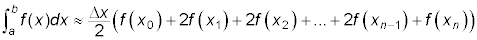where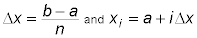## Simpson’s Rule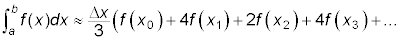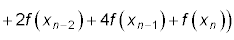where n is even and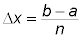## Special limit formulas

Many people first encounter the following limits in a Calculus textbook when trying to prove the derivative formulas for the sine function and the cosine function. These results aren’t immediately obvious and actually take a bit of work to justify. Any calculus text should provide more explanation if you’re interested in seeing it!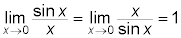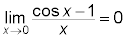## Derivative and integration formulas for hyperbolic functions

The hyperbolic functions are certain combinations of the exponential functions ex and ex. These functions occur often enough in differential equations and engineering that they’re typically introduced in a Calculus course. Some of the real-life applications of these functions relate to the study of electric transmission and suspension cables.